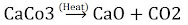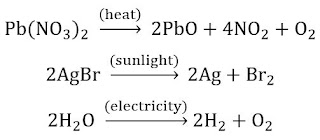# NCERT Solutions for Class 10 Science Chapter 1 Chemical Reactions and Equations

You can get Chapter 1 Chemical Reactions and Equations NCERT Solutions for Class 10 Science on this page which will make learning and understanding more easier. These NCERT Solutions for Class 10 are curated by our expert teachers who have vast experience of teaching students. Through these NCERT Solutions, you can track your progress anytime and provide complete assistance.## Chapter 1 Chemical Reactions and Equations Class 10 Science NCERT Solutions

NCERT In Text Questions

Page No: 6

1. Why should a magnesium ribbon be cleaned before burning in air?

Solution

When stored, magnesium reacts with oxygen to form a layer of magnesium oxide on its surface. Therefore, before burning it in air, it is cleaned by a sand paper which remove the protective layer of magnesium oxide from the surface of magnesium ribbon, so that it can easily burn.

2. Write the balanced equation for the following chemical reactions.
(i) Hydrogen + Chlorine → Hydrogen chloride
(ii) Barium chloride + Aluminium sulphate → Barium sulphate + Aluminium chloride
(iii) Sodium + Water → Sodium hydroxide + Hydrogen

Solution

(i) H2 (g) + Cl2 (g) → 2HCl (g)

(ii) 3BaCl2 (s) + Al2(SO4)3 (s) → 3BaSO4 (s) + 2AlCl3 (s)

(iii) 2Na(s) + 2H2O (l) → 2NaOH (aq) + H2 (g)

3. Write a balanced chemical equation with state symbols for the following reactions.
(i) Solutions of barium chloride and sodium sulphate in water react to give insoluble barium sulphate and the solution of sodium chloride.
(ii) Sodium hydroxide solution (in water) reacts with hydrochloric acid solution (in water) to produce sodium chloride solution and water.

Solution

(i) BaCl2 (aq) + Na2SO4 (aq) → BaSO4 (s) + 2NaCl (aq)

(ii) NaOH (aq) + HCl (aq) → NaCL (aq) + H2O (l)

Page No: 10

1. A solution of a substance ‘X’ is used for white washing.
(i) Name the substance ‘X’ and write its formula.
(ii) Write the reaction of the substance ‘X’ named in (i) above with water.

Solution

(i) The substance ‘X’ is calcium oxide. Its chemical formula is CaO.

(ii) Calcium oxide reacts vigorously with water to form calcium hydroxide (slaked lime).
CaO (s)  + H2O (l) → Ca(OH)2 (aq)
Calcium Oxide (Quick Lime) + Water → Calcium Hydroxide (Slaked Lime)

2. Why is the amount of gas collected in one of the test tubes in Activity 1.7 double of the amount collected in the other? Name this gas.

Solution

When electric current is passed through water it decomposes into its constituent components, i.e. hydrogen and oxygen gases. During electrolysis of water hydrogen gas collected in one test tube and is twice in volume than oxygen collected in another test tube. This is because a water molecule is formed by the combination of hydrogen and oxygen in the ratio of 2:1.

Page No: 13

1. Why does the colour of copper sulphate solution change when an iron nail is dipped in it?

Solution

An iron is more reactive than copper thus it displaces copper from copper sulphate solution.
Fe (s) + CuSO4 (aq) → FeSO4 (aq) + Cu (s)
(Blue)                                      (Green)
Thus, concentration of copper sulphate decreases while concentration of ferrous sulphate increases. As a result, colour changes from blue to green.

2. Give an example of a double displacement reaction other than the one given in Activity 1.10.

Solution

2Kl(aq.) + Pb(No3)2(aq.) → 2KNO3(aq.) + PbI2(s)
When lead nitrate reacts with potassium iodide, potassium nitrate and lead iodide are formed. In this reaction both the reactants exchange their ions after reaction. This type of reaction is known as double displacement reaction.

3. Identify the substances that are oxidised and the substances that are reduced in the following reactions.
(i) 4Na (s) + O2 (g) → 2Na2O (s)
(ii) CuO (s) + H2 (g) → Cu (s) + H2O (l)

Solution

(i) Sodium (Na) is oxidised as it gains oxygen and oxygen gets reduced.

(ii) Copper oxide (CuO) is reduced to copper (Cu) while hydrogen (H2) gets oxidised to water (H2O).

Exercises

1. Which of the statements about the reaction below are incorrect?
2PbO (s) + C (s) → 2Pb (s) + CO2 (g)
(b) Carbon dioxide is getting oxidised.
(c) Carbon is getting oxidised.
(d) Lead oxide is getting reduced.

(i) (a) and (b)
(ii) (a) and (c)
(iii) (a), (b) and (c)
(iv) all

Solution

(i) (a) and (b)

2. Fe2O3 + 2Al → Al2O3 + 2Fe
The above reaction is an example of a
(a) combination reaction.
(b) double displacement reaction.
(c) decomposition reaction.
(d) displacement reaction.

Solution

(d) displacement reaction.

3. What happens when dilute hydrochloric acid is added to iron filings? Tick the correct answer.
(a) Hydrogen gas and iron chloride are produced.
(b) Chlorine gas and iron hydroxide are produced.
(c) No reaction takes place.
(d) Iron salt and water are produced.

Solution

(a) Hydrogen gas and iron chloride are produced.

4. What is a balanced chemical equation? Why should chemical equations be balanced?

Solution

An equation in which the number of atoms of each element is equal on the reactant side and product side is called balanced equation. According to the law of conservation of mass, total mass of the elements present in the reactants must be equal to the total mass of the elements present in products. Thus, a chemical equation should be balanced always.

5. Translate the following statements into chemical equations and then balance them.
(a) Hydrogen gas combines with nitrogen to form ammonia.
(b) Hydrogen sulphide gas burns in air to give water and sulphur dioxide.
(c) Barium chloride reacts with aluminium sulphate to give aluminium chloride and a precipitate of barium sulphate.
(d) Potassium metal reacts with water to give potassium hydroxide and hydrogen gas.

Solution

(a) 3H2 (g) + N2 (g) → 2NH3 (g)

(b) 2H2S (g) + 3O2 (g) → 2H2O (l) + 2SO2 (g)

(c) 3BaCl2 (aq) + Al2(SO4)3 (aq) → 2AlCl3 (aq) + 3BaSO4 (s)

(d) 2K (s) + 2H2O (l) → 2KOH (aq) + H2 (g)

6. Balance the following chemical equations.
(i) HNO3 + Ca(OH)2 → Ca(NO3)2 + H2O
(ii) NaOH + H2SO4 → Na2SO4 + H2O
(iii) NaCl + AgNO3 → AgCl + NaNO3
(iv) BaCl2 + H2SO4 → BaSO4 + HCl

Solution

(i) 2HNO3 + Ca(OH)2 → Ca(NO3)2 + 2H2O
(ii) 2NaOH + H2SO4 → Na2SO4 + 2H2O
(iii) NaCl + AgNO3 → AgCl + NaNO3
(iv) BaCl2 + H2SO4 → BaSO4 + 2HCl

7. Write the balanced chemical equations for the following reactions.
(a) Calcium hydroxide + Carbon dioxide → Calcium carbonate + Water
(b) Zinc + Silver nitrate → Zinc nitrate + Silver
(c) Aluminium + Copper chloride → Aluminium chloride + Copper
(d) Barium chloride + Potassium sulphate → Barium sulphate + Potassium chloride

Solution

(a) Ca(OH)2 + CO2 → CaCO3 + H2O

(b) Zn + 2AgNO3 → Zn(NO3)2 + 2Ag

(c) 2Al + 3CuCl2 → 2AlCl3 + 3Cu

(d) BaCl2 + K2SO4 → BaSO4 + 2KCl

8. Write the balanced chemical equation for the following and identify the type of reaction in each case.
(a) Potassium bromide (aq) + Barium iodide (aq)­ → Potassium iodide (aq) + Barium bromide(s)
(b) Zinc carbonate (s) → Zinc oxide (s) + Carbon dioxide (g)
(c) Hydrogen (g) + Chlorine (g) → Hydrogen chloride (g)
(d) Magnesium (s) + Hydrochloric acid (aq) → Magnesium chloride (aq) + Hydrogen (g)

Solution

(a) 2KBr (aq) + BaI2 (aq) → 2KI (aq) + BaBr2 (s): Double displacement reaction

(b) ZnCO3 (s) →  ZnO (s) + CO2 (g): Decomposition reaction

(c) H2 (g) + Cl2 (g) → 2HCl (g): Combination reaction

(d) Mg (s) + 2HCl (aq) → MgCl2 (aq) + H2 (g) : Displacement Reaction

9. What does one mean by exothermic and endothermic reactions? Give examples.

Solution

Chemical reactions that release energy in the form of heat, light, or sound are called exothermic reactions.
Example: C (g) + O2 (g) → CO2 + Heat Energy

Reactions that absorb energy or require energy in order to proceed are called endothermic reactions.
Example:10. Why is respiration considered an exothermic reaction? Explain.

Solution

During respiration glucose is broken into water and carbon dioxide and energy is released. That is why it is considered as an exothermic reaction.
C6H12O6 (aq) + 6O2 (g) → 6CO2 (g) + 6H2O (l) + Energy

11. Why are decomposition reactions called the opposite of combination reactions? Write equations for these reactions.

Solution

During decomposition reaction, a single reactant decompose to form two or more products, whereas in combination two or more reactants react to form a single product, example,
N2 + 3H2 → 2NH3 (Combination reaction)(Decomposition reaction)

12. Write one equation each for decomposition reactions where energy is supplied in the form of heat, light or electricity.

Solution13. What is the difference between displacement and double displacement reactions? Write equations for these reactions.

Solution

In Displacement reaction, an element which is more reactive displaces the other element which is less reactive from its salt solution.
For Example: CuSo4 (aq) + Zn (s) → ZnSO4 (aq) + Cu (s)
In Double displacement reaction, exchange of ions between two reactants takes place.
For Example: Na2SO4 (aq) + BaCl2 (aq) → BaSO4 (s) + 2NaCl (aq)

14. In the refining of silver, the recovery of silver from silver nitrate solution involved displacement by copper metal. Write down the reaction involved.

Solution

2AgNO3 (aq) + Cu (s) → Cu(NO3)2 (aq) + 2Ag (s)
Silver Nitrate + Copper → Copper Nitrate + Silver

15. What do you mean by a precipitation reaction? Explain by giving examples.

Solution

Those reactions which produce an insoluble product or precipitate are known as precipitation reaction.
For example: Na2CO3 (aq) + CaCl2 (aq) → CaCO3 (s) + 2NaCl (aq)
Sodium Carbonate + Calcium Chloride → Calcium Carbonate + Sodium Chloride
In this reaction, calcium carbonate is obtained as a precipitate. Hence, it is a precipitation reaction.

16. Explain the following in terms of gain or loss of oxygen with two examples each.
(a) Oxidation
(b) Reduction

Solution

(a) Oxidation: The reaction in which gain of oxygen takes place is called oxidation.
Example, C + O2 → CO2 [C is oxidised to CO2]
N2 + O2 → 2NO [N2 is oxidised to NO]

(b) Reduction: The reaction in which loss of oxygen takes place is called reduction.
Example, C + H2O → CO + H2 [H2O is reduced to H2]
CuO + H2 → Cu + H2O [CuO is reduced to Cu]

17. A shiny brown-coloured element ‘X’ on heating in air becomes black in colour. Name the element ‘X’ and the black coloured compound formed.

Solution

The shiny brown coloured element 'X'is copper. When it is heated in air, it becomes black due to deposit of copper oxide.18. Why do we apply paint on iron articles?

Solution

To prevent iron articles coming in contact with moisture present in air which cause rusting, we apply paint on it.

19. Oil and fat containing food items are flushed with nitrogen. Why?

Solution

When fats and oils are oxidised, they become rancid causing a change in smell and taste. So, food items containing oil and fat are flushed with nitrogen which prevents them to get oxidized and becoming rancid.

20. Explain the following terms with one example each.
(a) Corrosion
(b) Rancidity

Solution

(a) Corrosion: It is the process in which metals are slowly eaten up by the action of air moisture or chemicals. For example rusting is a form of corrosion in which iron is eaten up by the action of air and moisture and a reddish brown coating of iron oxide is formed as shown in the following chemical reaction.
2Fe + 3H2O → Fe2O3 + 3H2

(b) Rancidity: When the substance containing oils and fats are exposed to air they get oxidised and become rancid due to which their smell, taste and colour change. This process is known as rancidity. For example when a when butter is kept open for a long time then its smell and taste gets changed.